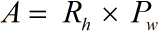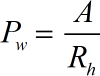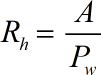Request a Tool

Hydraulic Radius Calculator is useful in calculating the hydraulic radius by putting the values Area of Section Flow (A) and Wetted Perimeter (Pw) in respective boxes.

Area
0

Formula• A = Area of Section Flow
• Pw = Wetted Perimeter

Defination / Uses

The ratio of the cross-sectional area of a conduit or stream channel through which a fluid is flowing to the inner perimeter of the conduit or stream channel is known as hydraulic radius.

Wetted Perimeter
0

Formula• Pw = Wetted Perimeter
• A = Area of Section Flow

Defination / Uses

The ratio of the cross-sectional area of a conduit or stream channel through which a fluid is flowing to the inner perimeter of the conduit or stream channel is known as hydraulic radius.

0

Formula• Pw = Wetted Perimeter
• A = Area of Section Flow

Defination / Uses

The ratio of the cross-sectional area of a conduit or stream channel through which a fluid is flowing to the inner perimeter of the conduit or stream channel is known as hydraulic radius.

How to use hydraulic radius calculator?

There are only two basic steps.

• First select the option from dropdown. What you need to calculate.
• Then add the other values in their corresponding fields.

Thats it! You will get your answer in less than a second. Use upper given formula in case of manual calculation.

The Hydraulic Radius concept states that the higher the ratio, the more efficient the channel is at transmitting water. The hydraulic radius is a measurement of the efficiency of a channel. By entering the numbers Area of Section Flow (A) and Wetted Perimeter (Pw) in the appropriate boxes, our online sophisticated Hydraulic Radius Calculator can calculate the hydraulic radius. The hydraulic radius, R, is defined as the ratio of the flow's cross sectional area, A, to the channel's wetted perimeter, P. For quick calculation use our weetools. No sign-up, registration OR captcha is required to use this tool.